# The DISCRIM Procedure

### PROC DISCRIM Statement

• PROC DISCRIM <options>;

The PROC DISCRIM statement invokes the DISCRIM procedure. Table 35.1 summarizes the options available in the PROC DISCRIM statement.

Table 35.1: Options Available in the PROC DISCRIM Statement

Option

Description

Input Data Sets

Specifies input SAS data set

Specifies input SAS data set to classify

Output Data Sets

Specifies output statistics data set

Specifies output data set with classification results

Specifies output data set with cross validation results

Specifies output data set with densities

Outputs discriminant scores to the OUT= data set

Specifies output data set with TEST= results

Specifies output data set with TEST= densities

Method Details

Specifies parametric or nonparametric method

Specifies whether to pool the covariance matrices

Specifies the singularity criterion

Specifies significance level homogeneity test

Specifies the minimum threshold for classification

Nonparametric Methods

Specifies k value for k nearest neighbors

Specifies proportion, p, for computing k

Specifies radius for kernel density estimation

Specifies a kernel density to estimate

Specifies metric in for squared distances

Canonical Discriminant Analysis

Performs canonical discriminant analysis

Specifies a prefix for naming the canonical variables

Specifies the number of canonical variables

Resubstitution Classification

Displays the classification results

Displays the misclassified observations

Suppresses the classification

Displays the classification results of TEST=

Displays the misclassified observations of TEST=

Cross Validation Classification

Displays the cross validation results

Displays the misclassified cross validation results

Specifies cross validation

Control Displayed Output

Displays all output

Displays univariate statistics

Displays between correlations

Displays between covariances

Displays between SSCPs

Displays squared Mahalanobis distances

Displays formulas in destinations other than LISTING

Displays multivariate ANOVA results

Suppresses all displayed output

Displays pooled correlations

Displays pooled covariances

Displays posterior probability error-rate estimates

Displays pooled SSCPs

Suppresses some displayed output

Displays simple descriptive statistics

Displays standardized class means

Displays total correlations

Displays total covariances

Displays total SSCPs

Displays within correlations

Displays within covariances

Displays within SSCPs

ALL

activates all options that control displayed output. When the derived classification criterion is used to classify observations, the ALL option also activates the POSTERR option.

ANOVA

displays univariate statistics for testing the hypothesis that the class means are equal in the population for each variable.

BCORR

displays between-class correlations.

BCOV

displays between-class covariances. The between-class covariance matrix equals the between-class SSCP matrix divided by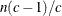, where n is the number of observations and c is the number of classes. You should interpret the between-class covariances in comparison with the total-sample and within-class covariances, not as formal estimates of population parameters.

BSSCP

displays the between-class SSCP matrix.

CANONICAL
CAN

performs canonical discriminant analysis.

CANPREFIX=name

specifies a prefix for naming the canonical variables. By default, the names are Can1, Can2, …, Cann. If you specify CANPREFIX=ABC, the components are named ABC1, ABC2, ABC3, and so on. The number of characters in the prefix, plus the number of digits required to designate the canonical variables, should not exceed 32. The prefix is truncated if the combined length exceeds 32.

The CANONICAL option is activated when you specify either the NCAN= or the CANPREFIX= option. A discriminant criterion is always derived in PROC DISCRIM. If you want canonical discriminant analysis without the use of discriminant criteria, you should use PROC CANDISC.

CROSSLIST

displays the cross validation classification results for each observation.

CROSSLISTERR

displays the cross validation classification results for misclassified observations only.

CROSSVALIDATE

specifies the cross validation classification of the input DATA= data set. When a parametric method is used, PROC DISCRIM classifies each observation in the DATA= data set by using a discriminant function computed from the other observations in the DATA= data set, excluding the observation being classified. When a nonparametric method is used, the covariance matrices used to compute the distances are based on all observations in the data set and do not exclude the observation being classified. However, the observation being classified is excluded from the nonparametric density estimation (if you specify the R= option) or the k nearest neighbors (if you specify the K= or KPROP= option) of that observation. The CROSSVALIDATE option is set when you specify the CROSSLIST, CROSSLISTERR, or OUTCROSS= option. With these options, cross validation information is displayed or output in addition to the usual resubstitution classification results. Cross validation classification results are written to the OUTCROSS= data set, and resubstitution classification results are written to the OUT= data set.

DATA=SAS-data-set

specifies the data set to be analyzed. The data set can be an ordinary SAS data set or one of several specially structured data sets created by SAS/STAT procedures. These specially structured data sets include TYPE=CORR, TYPE=COV, TYPE=CSSCP, TYPE=SSCP, TYPE=LINEAR, TYPE=QUAD, and TYPE=MIXED. The input data set must be an ordinary SAS data set if you specify METHOD=NPAR. If you omit the DATA= option, the procedure uses the most recently created SAS data set.

DISTANCE
MAHALANOBIS

displays the squared Mahalanobis distances between the group means, F statistics, and the corresponding probabilities of greater Mahalanobis squared distances between the group means. The squared distances are based on the specification of the POOL= and METRIC= options.

FORMULA

displays formulas in destinations other than LISTING. See the section Formulas for more information.

K=k

specifies a k value for the k-nearest-neighbor rule. An observationis classified into a group based on the information from the k nearest neighbors of. Do not specify the K= option with the KPROP= or R= option.

KPROP=p

specifies a proportion, p, for computing the k value for the k-nearest-neighbor rule: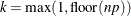, where n is the number of valid observations. When there is a FREQ statement, n is the sum of the FREQ variable for the observations used in the analysis (those without missing or invalid values). An observationis classified into a group based on the information from the k nearest neighbors of. Do not specify the KPROP= option with the K= or R= option.

KERNEL=BIWEIGHT | BIW
KERNEL=EPANECHNIKOV | EPA
KERNEL=NORMAL | NOR
KERNEL=TRIWEIGHT | TRI
KERNEL=UNIFORM | UNI

specifies a kernel density to estimate the group-specific densities. You can specify the KERNEL= option only when the R= option is specified. The default is KERNEL=UNIFORM.

LIST

displays the resubstitution classification results for each observation. You can specify this option only when the input data set is an ordinary SAS data set.

LISTERR

displays the resubstitution classification results for misclassified observations only. You can specify this option only when the input data set is an ordinary SAS data set.

MANOVA

displays multivariate statistics for testing the hypothesis that the class means are equal in the population.

METHOD=NORMAL | NPAR

determines the method to use in deriving the classification criterion. When you specify METHOD=NORMAL, a parametric method based on a multivariate normal distribution within each class is used to derive a linear or quadratic discriminant function. The default is METHOD=NORMAL. When you specify METHOD=NPAR, a nonparametric method is used and you must also specify either the K= or R= option.

METRIC=DIAGONAL | FULL | IDENTITY

specifies the metric in which the computations of squared distances are performed. If you specify METRIC=FULL, then PROC DISCRIM uses either the pooled covariance matrix (POOL=YES) or individual within-group covariance matrices (POOL=NO) to compute the squared distances. If you specify METRIC=DIAGONAL, then PROC DISCRIM uses either the diagonal matrix of the pooled covariance matrix (POOL=YES) or diagonal matrices of individual within-group covariance matrices (POOL=NO) to compute the squared distances. If you specify METRIC=IDENTITY, then PROC DISCRIM uses Euclidean distance. The default is METRIC=FULL. When you specify METHOD=NORMAL, the option METRIC=FULL is used.

NCAN=number

specifies the number of canonical variables to compute. The value of number must be less than or equal to the number of variables. If you specify the option NCAN=0, the procedure displays the canonical correlations but not the canonical coefficients, structures, or means. Let v be the number of variables in the VAR statement, and let c be the number of classes. If you omit the NCAN= option, only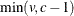canonical variables are generated. If you request an output data set (OUT=, OUTCROSS=, TESTOUT=), v canonical variables are generated. In this case, the last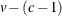canonical variables have missing values.

The CANONICAL option is activated when you specify either the NCAN= or the CANPREFIX= option. A discriminant criterion is always derived in PROC DISCRIM. If you want canonical discriminant analysis without the use of discriminant criterion, you should use PROC CANDISC.

NOCLASSIFY

suppresses the resubstitution classification of the input DATA= data set. You can specify this option only when the input data set is an ordinary SAS data set.

NOPRINT

suppresses the normal display of results. Note that this option temporarily disables the Output Delivery System (ODS); see Chapter 20: Using the Output Delivery System, for more information.

OUT=SAS-data-set

creates an output SAS data set containing all the data from the DATA= data set, plus the posterior probabilities and the class into which each observation is classified by resubstitution. When you specify the CANONICAL option, the data set also contains new variables with canonical variable scores. See the section OUT= Data Set for more information.

OUTCROSS=SAS-data-set

creates an output SAS data set containing all the data from the DATA= data set, plus the posterior probabilities and the class into which each observation is classified by cross validation. When you specify the CANONICAL option, the data set also contains new variables with canonical variable scores. See the section OUT= Data Set for more information.

OUTD=SAS-data-set

creates an output SAS data set containing all the data from the DATA= data set, plus the group-specific density estimates for each observation. See the section OUT= Data Set for more information.

OUTSTAT=SAS-data-set

creates an output SAS data set containing various statistics such as means, standard deviations, and correlations. When the input data set is an ordinary SAS data set or when TYPE=CORR, TYPE=COV, TYPE=CSSCP, or TYPE=SSCP, this option can be used to generate discriminant statistics. When you specify the CANONICAL option, canonical correlations, canonical structures, canonical coefficients, and means of canonical variables for each class are included in the data set. If you specify METHOD=NORMAL, the output data set also includes coefficients of the discriminant functions, and the output data set is TYPE=LINEAR (POOL=YES), TYPE=QUAD (POOL=NO), or TYPE=MIXED (POOL=TEST). If you specify METHOD=NPAR, this output data set is TYPE=CORR. This data set also holds calibration information that can be used to classify new observations. See the sections Saving and Using Calibration Information and OUT= Data Set for more information.

PCORR

displays pooled within-class correlations.

PCOV

displays pooled within-class covariances.

POOL=NO | TEST | YES

determines whether the pooled or within-group covariance matrix is the basis of the measure of the squared distance. If you specify POOL=YES, then PROC DISCRIM uses the pooled covariance matrix in calculating the (generalized) squared distances. Linear discriminant functions are computed. If you specify POOL=NO, the procedure uses the individual within-group covariance matrices in calculating the distances. Quadratic discriminant functions are computed. The default is POOL=YES. The k-nearest-neighbor method assumes the default of POOL=YES, and the POOL=TEST option cannot be used with the METHOD=NPAR option.

When you specify METHOD=NORMAL, the option POOL=TEST requests Bartlett’s modification of the likelihood ratio test (Morrison 1976; Anderson 1984) of the homogeneity of the within-group covariance matrices. The test is unbiased (Perlman 1980). However, it is not robust to nonnormality. If the test statistic is significant at the level specified by the SLPOOL= option, the within-group covariance matrices are used. Otherwise, the pooled covariance matrix is used. The discriminant function coefficients are displayed only when the pooled covariance matrix is used.

POSTERR

displays the posterior probability error-rate estimates of the classification criterion based on the classification results.

PSSCP

displays the pooled within-class corrected SSCP matrix.

R=r

specifies a radius r value for kernel density estimation. With uniform, Epanechnikov, biweight, or triweight kernels, an observationis classified into a group based on the information from observationsin the training set within the radius r of—that is, the group t observationswith squared distance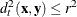. When a normal kernel is used, the classification of an observationis based on the information of the estimated group-specific densities from all observations in the training set. The matrix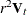is used as the group t covariance matrix in the normal-kernel density, where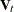is the matrix used in calculating the squared distances. Do not specify the K= or KPROP= option with the R= option. For more information about selecting r, see the section Nonparametric Methods.

SCORES<= prefix>

computes and outputs discriminant scores to the OUT= and TESTOUT= data sets with the default options METHOD=NORMAL and POOL=YES (or with METHOD=NORMAL, POOL=TEST, and a nonsignificant chi-square test). Otherwise, or if no OUT= or TESTOUT= data set is specified, this option is ignored. The scores are computed by a matrix multiplication of an intercept term and the raw data or test data by the coefficients in the linear discriminant function. One score variable is created for each level of the CLASS variable. By default, the variables are named "Sc_" followed by the formatted class level. You can specify SCORES=prefix to use a prefix other than "Sc_". The specifications SCORES and SCORES=Sc_ are equivalent.

SHORT

suppresses the display of certain items in the default output. If you specify METHOD=NORMAL, then PROC DISCRIM suppresses the display of determinants, generalized squared distances between-class means, and discriminant function coefficients. When you specify the CANONICAL option, PROC DISCRIM suppresses the display of canonical structures, canonical coefficients, and class means on canonical variables; only tables of canonical correlations are displayed.

SIMPLE

displays simple descriptive statistics for the total sample and within each class.

SINGULAR=p

specifies the criterion for determining the singularity of a matrix, where 0< p < 1. The default is SINGULAR=1E–8.

Let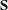be the total-sample correlation matrix. If the R square for predicting a quantitative variable in the VAR statement from the variables preceding it exceeds 1 – p, thenis considered singular. Ifis singular, the probability levels for the multivariate test statistics and canonical correlations are adjusted for the number of variables with R square exceeding 1 – p.

Let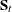be the group t covariance matrix, and let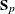be the pooled covariance matrix. In group t, if the R square for predicting a quantitative variable in the VAR statement from the variables preceding it exceeds 1 – p, thenis considered singular. Similarly, if the partial R square for predicting a quantitative variable in the VAR statement from the variables preceding it, after controlling for the effect of the CLASS variable, exceeds 1 – p, thenis considered singular.

If PROC DISCRIM needs to compute either the inverse or the determinant of a matrix that is considered singular, then it uses a quasi inverse or a quasi determinant. For details, see the section Quasi-inverse.

SLPOOL=p

specifies the significance level for the test of homogeneity. You can specify the SLPOOL= option only when POOL=TEST is also specified. If you specify POOL= TEST but omit the SLPOOL= option, PROC DISCRIM uses 0.10 as the significance level for the test.

STDMEAN

displays total-sample and pooled within-class standardized class means.

TCORR

displays total-sample correlations.

TCOV

displays total-sample covariances.

TESTDATA=SAS-data-set

names an ordinary SAS data set with observations that are to be classified. The quantitative variable names in this data set must match those in the DATA= data set. When you specify the TESTDATA= option, you can also specify the TESTCLASS , TESTFREQ , and TESTID statements. When you specify the TESTDATA= option, you can use the TESTOUT= and TESTOUTD= options to generate classification results and group-specific density estimates for observations in the test data set. Note that if the CLASS variable is not present in the TESTDATA= data set, the output will not include misclassification statistics.

TESTLIST

lists classification results for all observations in the TESTDATA= data set.

TESTLISTERR

lists only misclassified observations in the TESTDATA= data set but only if a TESTCLASS statement is also used.

TESTOUT=SAS-data-set

creates an output SAS data set containing all the data from the TESTDATA= data set, plus the posterior probabilities and the class into which each observation is classified. When you specify the CANONICAL option, the data set also contains new variables with canonical variable scores. See the section OUT= Data Set for more information.

TESTOUTD=SAS-data-set

creates an output SAS data set containing all the data from the TESTDATA= data set, plus the group-specific density estimates for each observation. See the section OUT= Data Set for more information.

THRESHOLD=p

specifies the minimum acceptable posterior probability for classification, where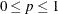. If the largest posterior probability of group membership is less than the THRESHOLD value, the observation is labeled as Other. The default is THRESHOLD=0. In some cases, you might want to specify a THRESHOLD= value slightly smaller than the desired p so that observations with posterior probabilities within rounding error of p are classified. For example, you can specify threshold=%sysevalf(0.5 - 1e-8) instead of THRESHOLD=0.5 so that observations with posterior probabilities within 1E–8 of 0.5 and larger are classified.

TSSCP

displays the total-sample corrected SSCP matrix.

WCORR

displays within-class correlations for each class level.

WCOV

displays within-class covariances for each class level.

WSSCP

displays the within-class corrected SSCP matrix for each class level.